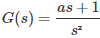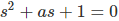# MCQs on Control Systems

##### Page 21 of 23. Go to page 1 2 3 4 5 6 7 8 9 10 11 12 13 14 15 16 17 18 19 20 21 22 23
01․ The transfer function of a plant is T(s) = 5/(s+5)(s² + 5 + 1). The second order approximation of T(s) using dominant pole concept is
1/(s+1)(s+5)
5/(s+1)(s+5)
1/(s² + 5 + 1)
5/(s² + 5 + 1)

Using dominant pole concept, T(s) = 5/(s+5)(s² + 5 + 1) T(s) = 5/5(s/5+1)(s² + 5 + 1) T(s) = 1/(s² + 5 + 1)

02․ The phase margin of a system having the loop transfer function G(s)H(s) = 2√3/s(s+1) is
45°
30°
60°
80°

The characteristic equation for given system is s² + s + 3.46 = 0 Phase margin ≈ 100*ζ Damping ratio = 0.27 Phase margin = 27° ≈ 30°

03․ A system has poles at 0.01 Hz, 1 Hz and 80 Hz; zeros at 5 Hz, 100 Hz and 200 Hz. The approximate phase of the system response at 20 Hz is
90°
180°
-90°
-180°

Using dominant pole technique, all the pole and zeros are insignificant except a pole at 0.01 Hz. Therefore, given system consists of only a pole at 0.01 Hz. Therefore, the approximate phase of the system is -90°.

04․ The Laplace transform of a transportation lag of 5 seconds is
e5s.
e-5s.
1/(s+5).
1/(s-5).

Dead time in real system is also known as transportation lag. Transfer function of transportation lag = e-Ts Therefore, transportation lag of 5 seconds can be written as e-5s.

05․ A property of phase lead compensation is that the
overshoot is increased.
bandwidth of closed loop system is reduced.
rise time of closed loop system is reduced.
gain margin is reduced.

1. 1. For sinusoidal input, the phase of controller output leads by tan-1 (ωTd).
2. 2. In terms of filtering property it acts as high pass filter.
3. 3. It reduces rise time.
4. 4. It increases bandwidth.
5. 5. It increases stability of the system.
6. 6. It reduces peak over shoot.

06․ Which of the following is the correct expression for the transfer function of an electrical RC phase lag compensating network?
RCS/(1+RCS)
RC/(1+RCS)
C/(1+RCS)
1/(1+RCS)

Phase lag compensator acts as a low pass filter. Therefore transfer function for lag compensator = 1/(1+RCS).

07․ The value of 'a' into give phase margin = 45o will be
0.707.
1.414.
1.18.
0.9.

Characteristic equation isNatural frequency ωn = 1 rad/s Phase margin = 100*ζ Damping ratio ζ = 45/100 = 0.45 2ζωn = a Therefore, a = 0.9

08․ A unity feedback control system has forward loop transfer function as e-Ts/s(s+1). Its phase value will be zero at frequency ω1. Which of the following equations should be satisfied by ω1?
ω1 = tan(Tω1)
ω1 = cot(Tω1)
1 = cot(ω1)
1 = tan(ω1)

Given transfer function = e-Ts/s(s+1) G(jω) = e-Tjω/jω(jω+1) ∠G(jω) = -ωT - π/2 - tan-1ω At ω = ω1;∠G(jω) = 0 -ω1T - π/2 - tan-1ω1 = 0 - tan-1ω1 = ω1T + π/2 - ω1 = -cot(Tω1) ω1 = cot(Tω1)

09․ In polar plots if the critical point '-1+j0' is enclosed then the system is --------
stable
unstable
marginally stable
critical stable

A point is said to be enclosed by a contour if it lies to the right side of the direction of the contour. A point is said to be encircled if the contour is a closed path. In polar plots if the critical point '-1+j0' is enclosed then the system is said to be unstable and if it not enclosed then the system is said to be stable.

10․ Resonant peak of a marginally stable system is
0
finite value
infinite
any of the above

Resonant peak is the maximum value of magnitude occurring at resonant frequency ωr. Resonant peak µr = 1/(2ζ√(1-ζ²)) For marginally stable system, damping ratio ζ = 0 Therefore, resonant peak = Infinity

<<<1920212223>>>